# Charge-Pump and Step-Up DC-DC Converter Solutions for Powering White LEDs in Series or Parallel Connections

### Abstract

White LEDs are popular for portable equipment LCD backlights because they are less complex, less expensive, and smaller than CCFL backlights. Portable equipment like PDAs, cellphones, and digital cameras require backlights because they are converting to color LCD displays. This article discusses charge-pump and inductor step-up converter solutions for powering white LEDs in series or parallel configurations. Regulated-voltage or regulated-current controllers are used. Trade-offs in size, efficiency, battery life, and LED-matching are discussed.

With the increasing popularity of color LCD displays in cell-phones, PDAs, and digital cameras, white LEDs are becoming popular illumination sources. While monochrome displays can use colored light sources such as electroluminescent back lights or colored LEDs, color displays require a white light source to properly display color.

There are two main methods for providing a white light source: white LEDs and CCFLs (cold-cathode fluorescent lamps). CCFLs have been used for years in notebook computers. However, because of their size, complexity, and cost advantages, white LEDs are becoming the preferred light source for small handheld devices.

White LEDs require a low DC voltage (between 3V and 4V), which means that simple inductor or capacitor-based circuits may be used for power. CCFLs, in contrast, require a high AC voltage (200VRMS to 500VRMS) and an expensive, bulky, transformer-based circuit for power (Figure 1).Figure 1. CCFL circuits require a transformer to deliver high voltage to the fluorescent tube.

The forward-voltage drop of red and green LEDs is typically 1.8V to 2.4V and low enough to be driven directly from a typical battery supply. White LEDs, however, typically have a forward-voltage drop of 3V to 4V and are more likely to need a separate power supply.

### Driving the LEDs

Luminous intensity increases with current through the LED. Full light output occurs around 20mA. Digital cameras and cellphones typically use two to three LEDs, while PDAs use three to six LEDs.

LEDs are driven in parallel or in series, as illustrated in Figure 2. A weakness of the parallel method is that LED current and brightness do not automatically match. The series method features inherent matching, but requires a higher supply voltage.Figure 2. Method A (LEDs in parallel) uses the lowest supply voltage, but method B (LEDs in series) gives the best matching.

Since the battery voltage of most handheld devices is too low for either method, a boost converter is needed. Charge-pump converters provide the smallest and lowest cost solution as they rely only on small capacitors for voltage conversion. However, charge-pump converters are only efficient when generating discrete multiples of their input voltages (e.g., 1.5x, 2x). Consequently, inductor-based converters are generally used in the LED series method. With inductor-based converters, higher step-up ratios are easily achieved and high efficiency is maintained over a wide range of input-to-output voltages.

### Driving Parallel LEDs

Figure 3 shows the three main methods of driving parallel connections of LEDs.

1. Independently regulate the current through each LED by using an existing supply.
2. Regulate the supply voltage only and rely on LED matching and series resistors for current matching.
3. Regulate current through one LED and rely on LED matching and series resistors for matching the remaining LEDs.Figure 3. Three methods of driving parallel LEDs are shown. A) Independently regulates the current through each LED. B) Regulates the output voltage and relies on series resistors to match currents. C) Regulates the current in one LED and relies on series resistors to match the remaining currents.

### Method A. Independently Regulate the Current Through Each LED

Integrated LED Current Regulator
If there is a supply voltage high enough to drive the forward drop of the LEDs, the only design issues are controlling the current and supplying the extra current to drive all LEDs at full brightness.

Figure 4 shows the MAX1916 used to drive three white LEDs at constant current, making that device a low-cost solution for matching LED brightness. Absolute current only needs to be set between the desired maximum brightness and the maximum rating of the LED; current matching must be good enough to maintain even display illumination. Typical current match is 0.3% with an absolute current accuracy of ±10%. Dropout voltage at each output is less than 410mV to maintain 20mA current. Thus, only 4.2V is needed to drive this circuit with 3.8V LEDs. The LED pin current is set to 230 times the current flowing into the SET pin. To supply the bias current to the SET pin, RSET is connected to a voltage greater than the 1.215V SET-pin bias voltage .Figure 4. The MAX1916 is available in a SOT23 package and provides 0.3% current matching.Figure 5. Three methods for adjusting the current in white LEDs with a MAX1916.

There are several methods of dynamically adjusting the brightness of the LEDs, as shown in Figure 5.

One method drives RSET with a DAC (Figure 5A). The LED current is a function of the DAC output minus the SET-pin bias voltage. DACs useful in this approach include the low-cost MAX5360–MAX5362/MAX5363–MAX5365 series in SOT23 packages.

A simple dimmer using several resistors driven by controller I/O pins is shown in Figure 5B. Toggle the pins between high (on) and tri-state (off) for the desired SET-pin current.

A final method drives the ENABLE pin with a logic-level PWM signal (Figure 5C). Many processors have ports that can provide a low-frequency PWM signal from 0 to 100% duty cycle. The response time of the MAX1916 ENABLE pin allows PWM operation up to around 2kHz.

Integrated Charge-Pump Boost Supply and Current Regulator
If an existing voltage supply is not available, a dedicated LED supply must be used. The low-cost MAX1574/MAX1575/MAX1576 charge-pump controllers combine boost-supply and current-regulator functions. These parts offer high output current, good current matching, adaptive mode switching for high efficiency, overvoltage protection, and up to eight LED drive pins.

Adaptive mode switching senses the input voltage and determines the most efficient step-up ratio (i.e., 1x, 1.5x, or 2x) to use. By using a serial pulse-code scheme, programmable dimming (as a percentage of the set current) is available through the DualMode™ enable pin.

Figure 6 shows a MAX1574 charge pump driving three LEDs at up to 180mA total current. The 1MHz switching rate allows use of small ceramic capacitors in the charge pump.

Figure 7 shows a MAX1576 charge pump driving two groups of four LEDs at up to 480mA total current. The flash group allows up to 100mA per LED. Each group has independent set current, serial pulse dimming, and 2-wire log dimming controls. With adaptive mode switching, average efficiency is 83% over the discharge curve of a single lithium battery (Figure 8). The MAX1576 is ideal for digital still-camera applications using LED flash.

The MAX1575 is a part variation that drives two groups of LEDs (four main LEDs and two sub-LEDs) at 120mA total output.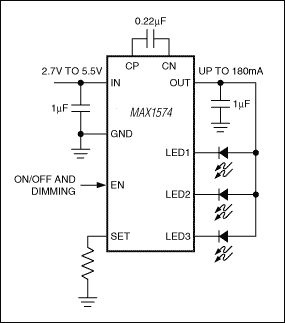Figure 6. MAX1574 charge pump with one group of LED current sources.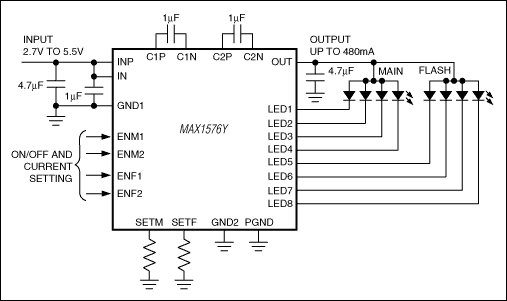Figure 7. MAX1576 charge pump with two groups of LED current sources.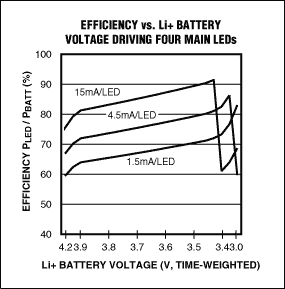Figure 8. MAX1576 efficiency at typical lithium battery voltages.

### Method B. Using a Supply with a Regulated Output Voltage

Like Method A, Method B is used when an existing voltage supply is available. Method B is very economical, but less accurate than Method A. As current is not regulated in Method B, absolute current through each LED must be kept between the desired maximum brightness and the maximum rating of the LED. Current matching must be good enough to maintain uniform illumination.

Referring to Method B in Figure 3 above, the current through any LED can be determined by subtracting the forward LED voltage (VD) from the output voltage of the supply (VOUT) and dividing by R:

 I = (VOUT - VD)/R (Eq. 1)

Figure 9A shows the I-V curves for two white LEDs taken from a typical handheld device. For the same current, the voltages across the diodes are not equal. Figure 9B shows the voltage difference between the LEDs as a function of current.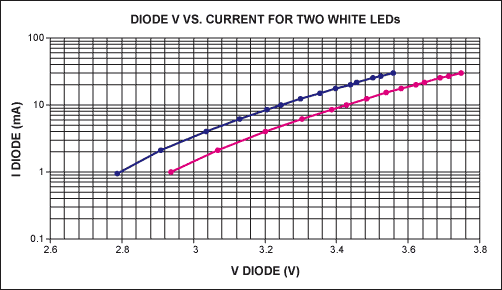Figure 9A. The I-V curves for two white LEDs taken from a typical handheld device.Figure 9B. The voltage difference between two white LEDs vs. current.

Mismatches in LED Current
To understand how the mismatch in forward voltages affects the current matching, one can take the ratio of the LED currents using Equation 1. For example, the ratio of I1 to I2 is given by:

 I1/I2 = R2/R1 ((VOUT - VD1)/(VOUT - VD2)) (Eq. 2)

If we simplify Equation 2 by assuming that R1 = R2 , Equation 2 reduces to:

 I1/I2 = (VOUT - VD1)/(VOUT - VD2) (Eq. 3)

As VOUT approaches very high values, Equation 3 approaches unity. Thus for better current matching, a higher VOUT is beneficial. R must increase in proportion to VOUT - VD to maintain constant current. The penalty for higher VOUT is more power dissipation in R. The trade-off is efficiency vs. current matching.

As an example, a 5V supply with a 3.60V LED leaves 1.40V for R. If that LED is replaced with a 3.42V LED, the voltage across R increases to nearly 1.58V, a 13% increase in LED current. Note that the LED voltage only changed by 5%. This example represents a reasonable trade-off between efficiency and matching.

Absolute Accuracy
Errors in absolute LED current for Method B in Figure 3 above can be calculated using Equation 1. Use graphs of VD vs. ID (Figure 9A) for the selected LED.

In Equation 1 substitute the desired operating current, I, the nominal voltage for VD at current I (from the graphs), and the selected VOUT. Solve for R. Take that R value and solve Equation 1 using the worst case VD from the LED data sheet. Be sure to allow for temperature variation of VD. This will result in the expected range of LED current. Be sure that it is less than the maximum rating for the LED.

Dimming Methods
LED current in Method B of Figure 3 may be adjusted by changing VOUT. This approach is not, however, recommended for shared supplies. Alternatively, MOSFETs can be used to switch resistors in parallel for a simple dimmer (Figure 10). However, this method quickly becomes expensive if many dimming levels are required. Consequently, the integrated approach of Figure 6 or the series-string approach (discussed below) should be considered.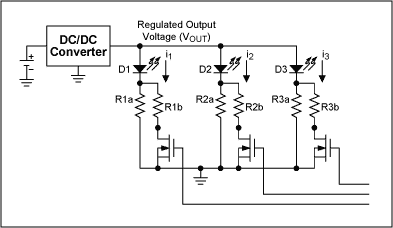Figure 10. Dimming with MOSFETs to switch resistors R1b to R3b in parallel with R1a to R3a.

### Method C. Using a Converter with a Regulated Output Current

Method C in Figure 3 shows a converter regulating current instead of voltage. In this design, the current through one of the LEDs is sensed across resistor R1 and regulated by the converter. The converter may be an inductor-based converter, a charge-pump converter, or a linear regulator.

The Equation for the LED current is the same as in Equation 1, and is repeated here for convenience:

 I = (VOUT - VD)/R (Eq. 4)

Unlike the case above, however, I1 instead of VOUT is regulated and given by:

 I1 = VFEEDBACK/R1 (Eq. 5)

Only one current is regulated, so differences in forward voltages of the remaining LEDs cause errors in currents, as was discussed above. Again, the solution is to increase R1 but in the modified manner illustrated in Figure 11.Figure 11. Current matching for the circuit of Method C in Figure 3 is improved by increasing R1A. R1B must remain constant for the current selected. R2 and R3 are set to R1A + R1B.

Since the current must remain constant, R1 is separated into R1A and R1B. R1B controls the current and R1A controls the extra output voltage for the desired current matching. Substitute R1 = R1A + R1B into Equation 4; substitute R1 = R1B into Equation 5. R2 and R3 are set to R1A + R1B to match the currents.

A variation of this last method is shown in Figure 12 with the MAX1910/MAX1912 charge pumps. A dimming input has been added and the sense resistor is shared with all LEDs to regulate total current. These controllers offer 1.5x and 2x step-up options for improved efficiency. Up to 120mA of output current is available, depending on the input voltage. See the data sheet for more information.Figure 12. MAX1910/MAX1912 application with a dimming control.

### Driving LEDs in Series

Driving white LEDs in series has the inherent key advantage of uniform brightness since the same current flows through each LED. There is a disadvantage to this design—it requires a higher voltage because the forward drops add together. Inductor-based converters are commonly used for this configuration because they are efficient at generating high voltages. When choosing this type of converter, the output voltage rating of the Lx pin must be considered.

A number of parts can support various numbers of series LEDs based on the Lx pin voltage as shown in Table 1. A guardband between the maximum rating of the Lx pin and the maximum voltage of the series string of LEDs is needed to allow for overvoltage shutdown.

Table 1. Part Selection for the Desired Number of LEDs in Series

 PART Lx PIN RATING (V) # SERIES LEDs PACKAGE MAX1848 14 3 8-SOT23 MAX1561/MAX1599 30 6 8-TDFN MAX8595Z/MAX8596Z 37 8 8-TDFN MAX8595X/MAX8596X 40 9 8-TDFN

The MAX8596Z, for example, is a switching regulator specifically designed to drive up to eight white LEDs in series (Figure 13). The device has a 2.6V to 5.5V input voltage range allowing the IC to be powered from a single lithium battery or three NiCd/NiMH batteries. The MAX8596Z comes in a space-saving 8-pin TDFN package. It features fast 1MHz PWM operation, thus allowing small external components. An overvoltage lockout threshold of 32V to 36V protects the IC in the event that an LED becomes open circuit. An additional device feature is high temperature derating. Output current is reduced above 42°C ambient to reduce dissipation in the LEDs.Figure 13. The MAX8596Z switching regulator drives up to eight white LEDs in series.

The LED current may be adjusted by driving the CTRL pin with any DC voltage or an unfiltered PWM signal. The CTRL pin voltages from 0.24V to 1.72V drive the LEDs from dim to full brightness. Above 1.72V, output current is clamped at the maximum. PWM signals may be used from 200Hz to 200kHz; the error amplifier and compensation capacitor act as a filter for the PWM signal so no input filtering is needed.

The efficiency of the MAX8596Z driving various numbers of LEDs is shown in Figure 14. Maximum efficiency is above 85%.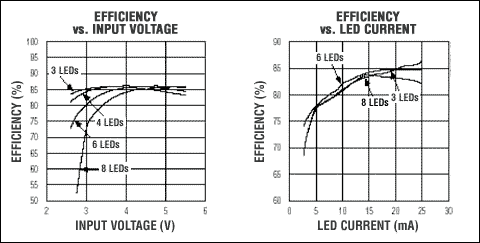Figure 14. MAX8596Z efficiency for the circuit of Figure 13.